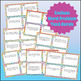# Add, Subtract, Multiply & Divide Task Cards ENDLESS Bundle: Fluency & Word ProbsSubject
Resource Type
Common Core Standards
Product Rating
File Type

Compressed Zip File

Share
Product Description

ENDLESS PRACTICE for all elementary addition, subtraction, multiplication, division and rounding! These 79,000 TASK CARDS practice all elementary computational skills using both word problems and simple fluency practice. They are broken into the following 79 sets with 1,000 task cards apiece:

Simple fluency practice:

✔ Adding 3 Numbers within 20

✔ Adding 10 to 2-Digit Numbers (1.NBT.5)

✔ Adding within 100 per 1.NBT.4

✔ Adding within 1,000 (2.NBT.7 and 3.NBT.2)

✔ Adding 4 2-Digit Numbers (2.NBT.6)

✔ Subtraction within 20

✔ Subtraction within 100

✔ Subtraction within 1,000

✔ Subtraction within 10,000

✔ Subtract Multiples of 10 (1.NBT.5)

✔ Subtract 10 from 2-Digit Numbers (1.NBT.5)

✔ Adding and Subtracting up to 20 (1.OA.6)

✔ Adding and Subtracting within 20 (2.OA.2)

✔ Adding and Subtracting 10 or 100 (2.NBT.8)

✔ Adding and Subtracting 10 (1.NBT.5)

✔ Adding and Subtracting within 100 (2.NBT.5)

✔ Adding and Subtracting within 1,000 (2.NBT.7)

✔ Adding and Subtracting up to 1,000 (3.NBT.2)

✔ Adding and Subtracting within 10,000

✔ Adding and Subtracting within 100,000

✔ Adding and Subtracting within 1,000,000 (4.NBT.4)

✔ Multiplication Facts 0 to 10

✔ Multiplication Facts 1 to 12

✔ Multiply 0 to 9 by Multiples of 10 (3.NBT.3)

✔ Multiply 1 Digit by 2 Digits

✔ Multiply 1 Digit by 3 Digits

✔ Multiply 2x2 and up to 4x1 Digits (4.NBT.5)

✔ Multiply 3x3 and up to 4x2 Digits (5.NBT.5)

✔ Three Digit Multiplication

✔ Two Digit Multiplication

✔ Multiply and Divide up to 100 (3.OA.7)

✔ Division Facts 0-10

✔ Division Facts 1-12

✔ Divide 3 Digits by 1 Digit

✔ Divide 4 Digits by 1 Digit

✔ Divide Up to 4 Digits by 1 Digit (4.NBT.6)

✔ Divide Up to 4 Digits by 2 Digits (5.NBT.6)

✔ Divide Within 100,000 (6.NS.2)

✔ Divide Within 1,000,000 (6.NS.2)

✔ Dividing with 2 Digit Divisors

✔ Dividing with 3 Digit Divisors

✔ All 4 Operations (5.NBT.7)

✔ All 4 Operations (6.NS.3)

Word problems:

✔ Adding within 100 per 1.NBT.4

✔ Adding within 1,000 (2.NBT.7 and 3.NBT.2)

✔ Subtracting up to 10

✔ Subtracting up to 20

✔ Subtracting up to 100

✔ Subtracting up to 1,000

✔ Subtracting up to 10,000

✔ Add and Subtract within 10

✔ Add and Subtract within 20 (1.OA.1)

✔ Add and Subtract within 100

✔ Add and Subtract within 1,000

✔ Add and Subtract within 10,000

✔ Multiplying 0 to 10

✔ Multiplying 1 to 12

✔ Three Digit Multiplication

✔ Two Digit Multiplication

✔ Multiplication and Division (0-10)

✔ Multiplication and Division (0-12)

✔ 2 Digit Multiplication and Division

✔ 3 Digit Multiplication and Division

✔ Multiply and Divide within 100 (3.OA.3)

✔ Dividing 0-10

✔ Dividing 0-12

✔ Dividing with 2 Digit Divisors

✔ Dividing with 3 Digit Divisors

Rounding:

✔ Rounding Decimals to Any Place (5.NBT.4)

✔ Rounding to 10 or 100 (3.NBT.1)

✔ Rounding to Any Place (4.NBT.3)

Task cards are great for a whole-class game, math centers/stations, fast finishers, extra practice, homework, scavenger hunt, quiz-quiz-trade, scoot, morning work, small groups, or review for test.

What’s included?

79,000 task cards printed 4 to a page

Four different sizes of student recording sheets

***************************************************************************

Check out my task cards, exit slips, worksheets, quizzes, tests, and more for grades K-6:

In Printable Format:

Kindergarten Math ULTIMATE Bundle, PDF Format

1st Grade Math ULTIMATE Bundle, PDF Format

2nd Grade Math ULTIMATE Bundle, PDF Format

3rd Grade Math ULTIMATE Bundle, PDF Format

4th Grade Math ULTIMATE Bundle, PDF Format

5th Grade Math ULTIMATE Bundle, PDF Format

6th Grade Math ULTIMATE Bundle, PDF Format

In Printable + Google Classroom Formats:

Total Pages
Included
Teaching Duration
Lifelong tool
Report this Resource\$49.99
List Price:
\$237.00
Bundle Price:
\$62.49
You Save:
\$187.01